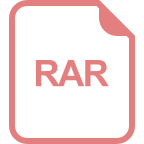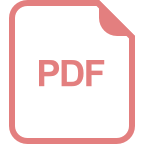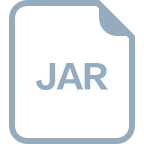# 像素坐标转相机坐标代码c++

### 回答1： 在使用像素坐标转相机坐标之前，需要先确定相机的内参矩阵和畸变参数。假设相机内参矩阵为K，畸变参数为D，像素坐标为(u, v)，则可以使用以下代码将像素坐标转换为相机坐标： ``` // 定义相机内参矩阵和畸变参数 cv::Mat K = (cv::Mat_<double>(3, 3) << fx, 0, cx, 0, fy, cy, 0, 0, 1); cv::Mat D = (cv::Mat_<double>(1, 5) << k1, k2, p1, p2, k3); // 像素坐标 cv::Point2d p(u, v); // 像素坐标转归一化坐标 cv::Point2d p_norm = (p - cv::Point2d(K.at<double>(0, 2), K.at<double>(1, 2))) / cv::Point2d(K.at<double>(0, 0), K.at<double>(1, 1)); // 去畸变 cv::Matx33d K_new = cv::Matx33d(K); cv::Vec4d D_new = cv::Vec4d(D); cv::Point2d p_undist = cv::fisheye::undistortPoint(p_norm, K_new, D_new); // 相机坐标 cv::Point3d p_cam(p_undist.x, p_undist.y, 1.0); ``` 其中，fx、fy、cx、cy、k1、k2、p1、p2、k3分别是相机内参矩阵和畸变参数中的元素。注意，这里使用的是OpenCV中的fisheye模块实现的畸变去除函数，如果相机不是鱼眼相机，则需要使用对应的畸变去除函数。 ### 回答2： // 像素坐标转相机坐标代码 C #include <iostream> #include <Eigen/Core> #include <Eigen/Geometry> Eigen::Vector3d pixel2Camera(const Eigen::Vector2d& pixel, const Eigen::Matrix3d& cameraMatrix, const Eigen::Vector4d& distortionCoefficients) { Eigen::Vector2d distortedPoint; // 应用畸变模型校正像素坐标 distortedPoint = (pixel - cameraMatrix(0, 2)) / cameraMatrix(0, 0); distortedPoint = (pixel - cameraMatrix(1, 2)) / cameraMatrix(1, 1); // 校正径向和切向畸变 double k1 = distortionCoefficients; double k2 = distortionCoefficients; double p1 = distortionCoefficients; double p2 = distortionCoefficients; double r2 = distortedPoint * distortedPoint + distortedPoint * distortedPoint; double k = 1 + k1 * r2 + k2 * r2 * r2; distortedPoint = distortedPoint * k + 2 * p1 * distortedPoint * distortedPoint + p2 * (r2 + 2 * distortedPoint * distortedPoint); distortedPoint = distortedPoint * k + p1 * (r2 + 2 * distortedPoint * distortedPoint) + 2 * p2 * distortedPoint * distortedPoint; // 通过相机内参矩阵将像素坐标转换为相机坐标 Eigen::Vector3d cameraPoint; cameraPoint = distortedPoint; cameraPoint = distortedPoint; cameraPoint = 1; cameraPoint = cameraMatrix.inverse() * cameraPoint; return cameraPoint; } int main() { // 示例用的相机内参矩阵 Eigen::Matrix3d cameraMatrix; cameraMatrix << 500, 0, 320, 0, 500, 240, 0, 0, 1; // 示例用的畸变系数 Eigen::Vector4d distortionCoefficients; distortionCoefficients << 0.1, -0.2, 0.01, -0.02; // 示例用的像素坐标 Eigen::Vector2d pixel(350, 250); // 调用像素坐标转相机坐标函数 Eigen::Vector3d cameraPoint = pixel2Camera(pixel, cameraMatrix, distortionCoefficients); // 打印结果 std::cout << "相机坐标：\n" << cameraPoint << std::endl; return 0; } ### 回答3： 像素坐标转相机坐标是计算机视觉中常用的问题，下面是一个简单的用C语言实现的代码示例： ```c #include <stdio.h> typedef struct { float x; float y; } PixelCoord; typedef struct { float x; float y; float z; } CamCoord; void pixelToCam(PixelCoord pixel, CamCoord* cam, float focal_length, float pixel_size, float image_width, float image_height) { cam->x = (pixel.x - image_width / 2) * pixel_size / focal_length; cam->y = (pixel.y - image_height / 2) * pixel_size / focal_length; cam->z = focal_length; } int main() { PixelCoord pixel = { 100, 200 }; // 指定像素坐标 float focal_length = 1000; // 焦距 float pixel_size = 0.01; // 像素大小 float image_width = 1920; // 图像宽度 float image_height = 1080; // 图像高度 CamCoord camera; pixelToCam(pixel, &camera, focal_length, pixel_size, image_width, image_height); printf("相机坐标：(%.2f, %.2f, %.2f)\n", camera.x, camera.y, camera.z); return 0; } ``` 在以上的代码中，定义了两个结构体`PixelCoord`和`CamCoord`来分别表示像素坐标和相机坐标。`pixelToCam`函数用于将像素坐标转换为相机坐标。最后的`main`函数中，给定了一个像素坐标，以及一些相机参数，调用`pixelToCam`函数将像素坐标转换为相机坐标，并输出结果。 以上仅是一个简单的实现示例，实际情况下，可能需要考虑更多的相机参数和图像处理步骤。如果需要更高级的像素坐标转相机坐标功能，建议使用计算机视觉库，如OpenCV，来简化开发过程。

## 相关推荐